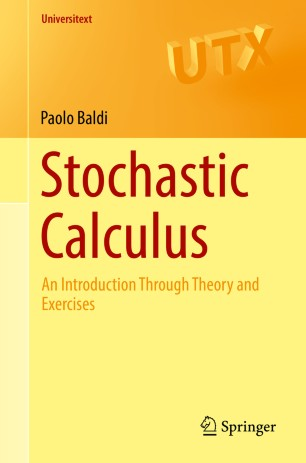# Stochastic Calculus

## An Introduction Through Theory and Exercises

• Paolo BaldiTextbook

Part of the Universitext book series (UTX)

1. Front Matter
Pages i-xiv
2. Paolo Baldi
Pages 1-29
3. Paolo Baldi
Pages 31-44
4. Paolo Baldi
Pages 45-84
5. Paolo Baldi
Pages 85-107
6. Paolo Baldi
Pages 109-150
7. Paolo Baldi
Pages 151-179
8. Paolo Baldi
Pages 181-213
9. Paolo Baldi
Pages 215-254
10. Paolo Baldi
Pages 255-303
11. Paolo Baldi
Pages 305-339
12. Paolo Baldi
Pages 341-364
13. Paolo Baldi
Pages 365-394
14. Paolo Baldi
Pages 395-435
15. Back Matter
Pages 437-627

### Introduction

This book provides a comprehensive introduction to the theory of stochastic calculus and some of its applications. It is the only textbook on the subject to include more than two hundred exercises with complete solutions.

After explaining the basic elements of probability, the author introduces more advanced topics such as Brownian motion, martingales and Markov processes. The core of the book covers stochastic calculus, including stochastic differential equations, the relationship to partial differential equations, numerical methods and simulation, as well as applications of stochastic processes to finance. The final chapter provides detailed solutions to all exercises, in some cases presenting various solution techniques together with a discussion of advantages and drawbacks of the methods used.

Stochastic Calculus will be particularly useful to advanced undergraduate and graduate students wishing to acquire a solid understanding of the subject through the theory and exercises. Including full mathematical statements and rigorous proofs, this book is completely self-contained and suitable for lecture courses as well as self-study.

### Keywords

stochastic calculus stochastic calculus exercises probability elements stochastic processes Brownian motion Brownian motion exercises conditional probability martingales martingales exercises Markov processes stochastic integral stochastic differential equations stochastic differential equations exercises PDE problems SDE approximation stochastic differential equation approximation stochastic calculus financial models stochastic calculus finance numerical simulation 60H10, 60H05, 60H30, 60G42, 60G44

#### Authors and affiliations

• Paolo Baldi
• 1
1. 1.Dipartimento di MatematicaUniversità di Roma “Tor Vergata”RomaItaly